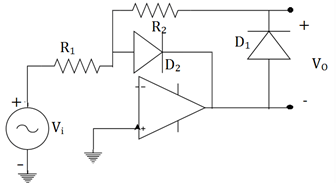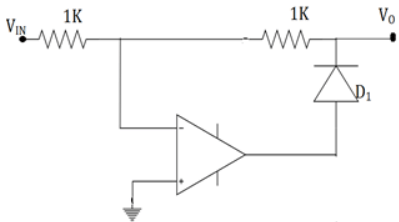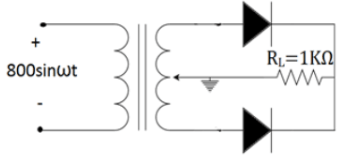Test: Half-Wave & Full-wave Rectifier

# Test: Half-Wave & Full-wave Rectifier

Test Description

## 20 Questions MCQ Test Analog Electronics | Test: Half-Wave & Full-wave Rectifier

Test: Half-Wave & Full-wave Rectifier for Electrical Engineering (EE) 2022 is part of Analog Electronics preparation. The Test: Half-Wave & Full-wave Rectifier questions and answers have been prepared according to the Electrical Engineering (EE) exam syllabus.The Test: Half-Wave & Full-wave Rectifier MCQs are made for Electrical Engineering (EE) 2022 Exam. Find important definitions, questions, notes, meanings, examples, exercises, MCQs and online tests for Test: Half-Wave & Full-wave Rectifier below.
Solutions of Test: Half-Wave & Full-wave Rectifier questions in English are available as part of our Analog Electronics for Electrical Engineering (EE) & Test: Half-Wave & Full-wave Rectifier solutions in Hindi for Analog Electronics course. Download more important topics, notes, lectures and mock test series for Electrical Engineering (EE) Exam by signing up for free. Attempt Test: Half-Wave & Full-wave Rectifier | 20 questions in 20 minutes | Mock test for Electrical Engineering (EE) preparation | Free important questions MCQ to study Analog Electronics for Electrical Engineering (EE) Exam | Download free PDF with solutions
 1 Crore+ students have signed up on EduRev. Have you?
Test: Half-Wave & Full-wave Rectifier - Question 1

### The diode in a half wave rectifier has a forward resistance RF. The voltage is Vmsinωt and the load resistance is RL. The DC current is given by _________

Detailed Solution for Test: Half-Wave & Full-wave Rectifier - Question 1

For a half wave rectifier, the IDC=IAVG=Im
I= Vmsinωt/(RF+RL)=Imsinωt
Im =Vm/ RF+RL So, IDC=Im/π=Vm/(RF+RL).

Test: Half-Wave & Full-wave Rectifier - Question 2

### The below figure arrives to a conclusion that _________Detailed Solution for Test: Half-Wave & Full-wave Rectifier - Question 2

The given op-amp is in inverting mode and this makes the output voltage to have a phase shift of 180°. The output voltage is now negative. So, the diode 1 is reverse biased and diode 2 is forward biased. Then output is clearly zero.

Test: Half-Wave & Full-wave Rectifier - Question 3

### What is the output as a function of the input voltage (for positive values) for the given figure. Assume it’s an ideal op-amp with zero forward drop (Di=0)Detailed Solution for Test: Half-Wave & Full-wave Rectifier - Question 3

When the input of the inverted mode op-amp is positive, the output is negative.
The diode is reverse biased. The input appears at the output.

Test: Half-Wave & Full-wave Rectifier - Question 4

In a half wave rectifier, the sine wave input is 50sin50t. If the load resistance is of 1K, then average DC power output will be?

Detailed Solution for Test: Half-Wave & Full-wave Rectifier - Question 4

The standard form of a sine wave is Vmsinωt. BY comparing the given information with this equation, Vm =50.
Power=Vm2/RL=50*50/1000=2.5V.

Test: Half-Wave & Full-wave Rectifier - Question 5

In a half wave rectifier, the sine wave input is 200sin300t. The average value of output voltage is?

Detailed Solution for Test: Half-Wave & Full-wave Rectifier - Question 5

Comparing with the standard equation, Vm=200V.
Average value is given by, Vavg=Vm/π.
So, 200/π=63.694.

Test: Half-Wave & Full-wave Rectifier - Question 6

Efficiency of a half wave rectifier is

Detailed Solution for Test: Half-Wave & Full-wave Rectifier - Question 6

Efficiency of a rectifier is the effectiveness to convert AC to DC. For half wave it’s 40.6%. It’s given by, Vout/Vin*100.

Test: Half-Wave & Full-wave Rectifier - Question 7

If peak voltage for a half wave rectifier circuit is 5V and diode cut in voltage is 0.7, then peak inverse voltage on diode will be?

Detailed Solution for Test: Half-Wave & Full-wave Rectifier - Question 7

PIV is the maximum reverse bias voltage that can be appeared across a diode in the given circuit, If the PIV rating is less than this value of breakdown of diode will occur. For a rectifier, PIV=Vm-Vd=5-0.7=4.3V.

Test: Half-Wave & Full-wave Rectifier - Question 8

Transformer utilisation factor of a half wave rectifier is _________

Detailed Solution for Test: Half-Wave & Full-wave Rectifier - Question 8

Transformer utilisation factor is the ratio of AC power delivered to load to the DC power rating. This factor indicates effectiveness of transformer usage by rectifier. For a half wave rectifier, it’s low and equal to 0.287.

Test: Half-Wave & Full-wave Rectifier - Question 9

If the input frequency of a half wave rectifier is 100Hz, then the ripple frequency will be_________

Detailed Solution for Test: Half-Wave & Full-wave Rectifier - Question 9

The ripple frequency of the output and input is same. This is because, one half cycle of input is passed and other half cycle is seized. So, effectively the frequency is the same.

Test: Half-Wave & Full-wave Rectifier - Question 10

Ripple factor of a half wave rectifier is_________(Im is the peak current and RL is load resistance)

Detailed Solution for Test: Half-Wave & Full-wave Rectifier - Question 10

The ripple factor of a rectifier is the measure of disturbances produced in the output. It’s the effectiveness of a power supply filter to reduce the ripple voltage. The ratio of ripple voltage to DC output voltage is ripple factor which is 1.21.

Test: Half-Wave & Full-wave Rectifier - Question 11

Efficiency of a centre tapped full wave rectifier is _________

Detailed Solution for Test: Half-Wave & Full-wave Rectifier - Question 11

Efficiency of a rectifier is the effectiveness to convert AC to DC. It’s obtained by taking ratio of DC power output to maximum AC power delivered to load. It’s usually expressed in percentage. For centre tapped full wave rectifier, it’s 81.2%.

Test: Half-Wave & Full-wave Rectifier - Question 12

A full wave rectifier supplies a load of 1KΩ. The AC voltage applied to diodes is 220V (rms). If diode resistance is neglected, what is the ripple voltage?

Detailed Solution for Test: Half-Wave & Full-wave Rectifier - Question 12

The ripple voltage is (Vϒ)RMS=ϒVDC /100.
VDC=0.636*VRMS* √2=0.636*220* √2=198V and ripple factor ϒ for full wave rectifier is 0.482.
Hence, (Vϒ)RMS=0.482*198 /100=0.954V.

Test: Half-Wave & Full-wave Rectifier - Question 13

A full wave rectifier delivers 50W to a load of 200Ω. If the ripple factor is 2%, calculate the AC ripple across the load.

Detailed Solution for Test: Half-Wave & Full-wave Rectifier - Question 13

We know that, PDC=VDC2/RL. So, VDC=(PDC*RL)1/2=100001/2=100V.
Here, ϒ=0.02
ϒ=VAC/VDC=VAC/100.So, VAC=0.02*100=2V.

Test: Half-Wave & Full-wave Rectifier - Question 14

A full wave rectifier uses load resistor of 1500Ω. Assume the diodes have Rf=10Ω, Rr=∞. The voltage applied to diode is 30V with a frequency of 50Hz. Calculate the AC power input.

Detailed Solution for Test: Half-Wave & Full-wave Rectifier - Question 14

The AC power input PIN=IRMS2(RF+Rr).
IRMS=Im/√2=Vm/(Rf+RL)√2=30/(1500+10)*1.414=13.5mA
So, PIN=(13.5*10-3)2*(1500+10)=275.2mW.

Test: Half-Wave & Full-wave Rectifier - Question 15

In a centre tapped full wave rectifier, RL=1KΩ and for diode Rf=10Ω. The primary voltage is 800sinωt with transformer turns ratio=2. The ripple factor will be _________​Detailed Solution for Test: Half-Wave & Full-wave Rectifier - Question 15

The ripple factor ϒ= [(IRMS/IAVG)2 – 1]1/2. IRMS =Im /√2=Vm/(Rf+RL)√2=200/1.01=198.
(Secondary line to line voltage is 800/2=400. Due to centre tap Vm=400/2=200)
IRMS=198/√2=140mA, IAVG=2*198/π=126mA. ϒ=[(140/126)2-1]1/2=0.48. So, ϒ=48%.

Test: Half-Wave & Full-wave Rectifier - Question 16

If input frequency is 50Hz for a full wave rectifier, the ripple frequency of it would be _________

Detailed Solution for Test: Half-Wave & Full-wave Rectifier - Question 16

In the output of the centre tapped rectifier, one of the half cycle is repeated. The frequency will be twice as that of input frequency. So, it’s 100Hz.

Test: Half-Wave & Full-wave Rectifier - Question 17

Transformer utilization factor of a centre tapped full wave rectifier is_________

Detailed Solution for Test: Half-Wave & Full-wave Rectifier - Question 17

Transformer utilisation factor is the ratio of AC power delivered to load to the DC power rating. This factor indicates effectiveness of transformer usage by rectifier. For a half wave rectifier, it’s low and equal to 0.693.

Test: Half-Wave & Full-wave Rectifier - Question 18

In the circuits given below, the correct full wave rectifier is _________

Detailed Solution for Test: Half-Wave & Full-wave Rectifier - Question 18

When the input is applied, a full wave rectifier should have a current flow. The flow should be in the same direction for both positive and negative half cycles. Only the third circuit satisfies the above condition.

Test: Half-Wave & Full-wave Rectifier - Question 19

If the peak voltage on a centre tapped full wave rectifier circuit is 5V and diode cut in voltage is 0.7. The peak inverse voltage on diode is_________

Detailed Solution for Test: Half-Wave & Full-wave Rectifier - Question 19

PIV is the maximum reverse bias voltage that can be appeared across a diode in the given circuit, if PIV rating is less than this value of breakdown of diode will occur. For a rectifier, PIV=2Vm-Vd = 10-0.7 = 9.3V.

Test: Half-Wave & Full-wave Rectifier - Question 20

In a centre tapped full wave rectifier, the input sine wave is 250sin100t. The output ripple frequency will be _________

Detailed Solution for Test: Half-Wave & Full-wave Rectifier - Question 20

The equation of sine wave is in the form Vmsinωt. So, by comparing we get ω=100. Frequency, f =ω/2=50Hz. The output of centre tapped full wave rectifier has double the frequency of inpu. Hence, fout = 100Hz.

## Analog Electronics

11 videos|57 docs|97 tests
 Use Code STAYHOME200 and get INR 200 additional OFF Use Coupon Code
Information about Test: Half-Wave & Full-wave Rectifier Page
In this test you can find the Exam questions for Test: Half-Wave & Full-wave Rectifier solved & explained in the simplest way possible. Besides giving Questions and answers for Test: Half-Wave & Full-wave Rectifier, EduRev gives you an ample number of Online tests for practice

## Analog Electronics

11 videos|57 docs|97 tests